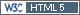## power_TOST VS statistical power of the ANOVA [Power / Sample Size]

In the results it is also evident that the post-hoc statistical power (power_TOST) is less than 80%, this is because the estimated point is greater than 105%, considering that the sample size was estimated expecting a difference less than / equal to 5%. However, the statistical power of the ANOVA (Power_80_20) supports that the sample size used in the study was adequate, since the values ​​are greater than 90%.

This is true?Ing. Helmut Schütz